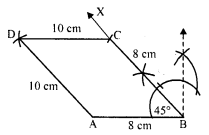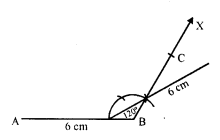# RD Sharma Class 8 Solutions Chapter 18 Practical Geometry Ex 18.3

In this chapter, we provide RD Sharma Class 8 Solutions Chapter 18 Practical Geometry Ex 18.3 for English medium students, Which will very helpful for every student in their exams. Students can download the latest RD Sharma Class 8 Solutions Chapter 18 Practical Geometry Ex 18.3 Maths pdf, free RD Sharma Class 8 Solutions Chapter 18 Practical Geometry Ex 18.3 book pdf download. Now you will get step by step solution to each question.

### RD Sharma Solutions for Class 8 Chapter 18 Practical Geometry Ex 18.3Download PDF

Question 1.
Construct a quadrilateral ABCD in which AB = 3.8 cm, BC = 3.4 cm, CD = 4.5 cm, AD = 5 cm and ∠B = 80°.
Solution:
Steps of construction :
(i) Draw a line segment AB = 3.8 cm.
(ii) At B, draw a ray BX making an angle of 80° and cut off BC = 3.4 cm.
(iii) With centre A and radius 5 cm and with centre C and radius 4.5 cm, draw arcs which intersect each other at D.Question 2.
Construct a quadrilateral ABCD given that AB = 8 cm, BC = 8 cm, CD = 10 cm, AD = 10 cm and ∠A = 45°.
Solution:
Steps of construction :
(i) Draw a line segment AB = 8 cm.(ii) At A, draw a ray AX making an angle of 45° and cut off BC = 8 cm.
(iii) With centre A and C and radius 10 cm, draw arcs intersecting each other at D.
Then ABCD is the required quadrilateral.

Question 3.
Construct a quadrilateral ABCD in which AB = 7.7 cm, BC = 6.8 cm, CD = 5.1 cm, AD = 3.6 cm and ∠C = 120°.
Solution:
Steps of construction :
(i) Draw a line segment BC = 6.8 cm.(ii) At C, draw a ray CX making an angle of 120° and cut off CD = 5.1 cm.
(iii) With centre B and radius 7.7 cm and with centre D and radius 3.6 cm draw arcs which intersect each other at A.
Then ABCD is the required quadrilateral.

Question 4.
Construct a quadrilateral ABCD in which AB = BC = 3 cm, AD = CD = 5 cm and ∠B = 120°
Solution:
Steps of construction :
(i) Draw a line segment AB = 3 cm.(ii) At B, draw a ray BX making an angle of 120° and cut off BC = 3 cm.
(iii) With centres A and C, and radius 5 cm, draw arcs intersecting each other at D.
Then ABCD is the required quadrilateral.

Question 5.
Construct a quadrilateral ABCD in which AB = 2.8 cm, BC = 3.1 cm, CD = 2.6 cm and DA = 3.3 cm and ∠A = 60°.
Solution:
Steps of construction :
(i) Draw a line segment AB = 2.8 cm.(ii) At A draw a ray AX making an angle of 60° and cut off AD = 3.3 cm.
(iii) With centre B and radius 3.1 cm and with centre D and radius 2.6 cm, draw arc which intersect each other at C.
(iv) Join CB and CD.
Then ABCD is the required quadrilateral.

Question 6.
Construct a quadrilateral ABCD in which AB = BC = 6 cm, AD = DC = 4.5 cm and ∠B = 120°.
Solution:
Steps of construction:
The construction is not possible to draw as arcs of radius 4.5 cm from A and C, do not intersect at any point.All Chapter RD Sharma Solutions For Class 8 Maths

—————————————————————————–

All Subject NCERT Exemplar Problems Solutions For Class 8

All Subject NCERT Solutions For Class 8

*************************************************

I think you got complete solutions for this chapter. If You have any queries regarding this chapter, please comment on the below section our subject teacher will answer you. We tried our best to give complete solutions so you got good marks in your exam.

If these solutions have helped you, you can also share rdsharmasolutions.in to your friends.